# RS Aggarwal Solutions for Class 7 Maths Exercise 15B Chapter 15 Properties of Triangles

RS Aggarwal Solutions for Class 7 Maths Exercise 15B Chapter 15 Properties of Triangles, Download PDF are given here. This exercise of RS Aggarwal Solutions for Class 7 Maths Chapter 15 Properties of Triangles, is prepared in such a way that students who are in 7th standard can grasp the concepts quickly and prepare themselves very well for exams to score high marks. The topics covered in this exercise are Exterior and Interior opposite angles and also Exterior angle property of a triangle. Students can score more marks in Maths by practising RS Aggarwal Solutions for Class 7 Maths.

## Download the PDF of RS Aggarwal Solutions For Class 7 Maths Chapter 15 Properties of Triangles – Exercise 15B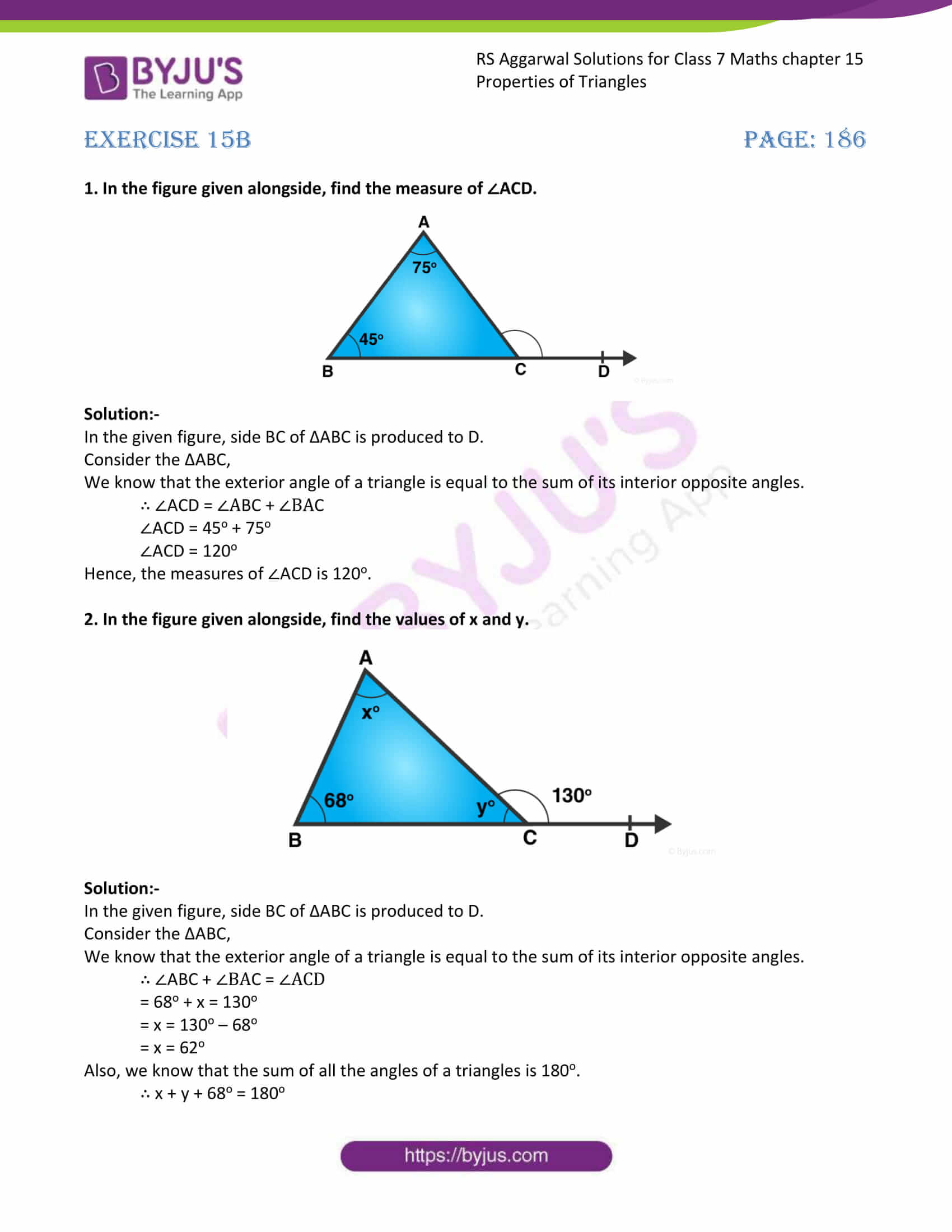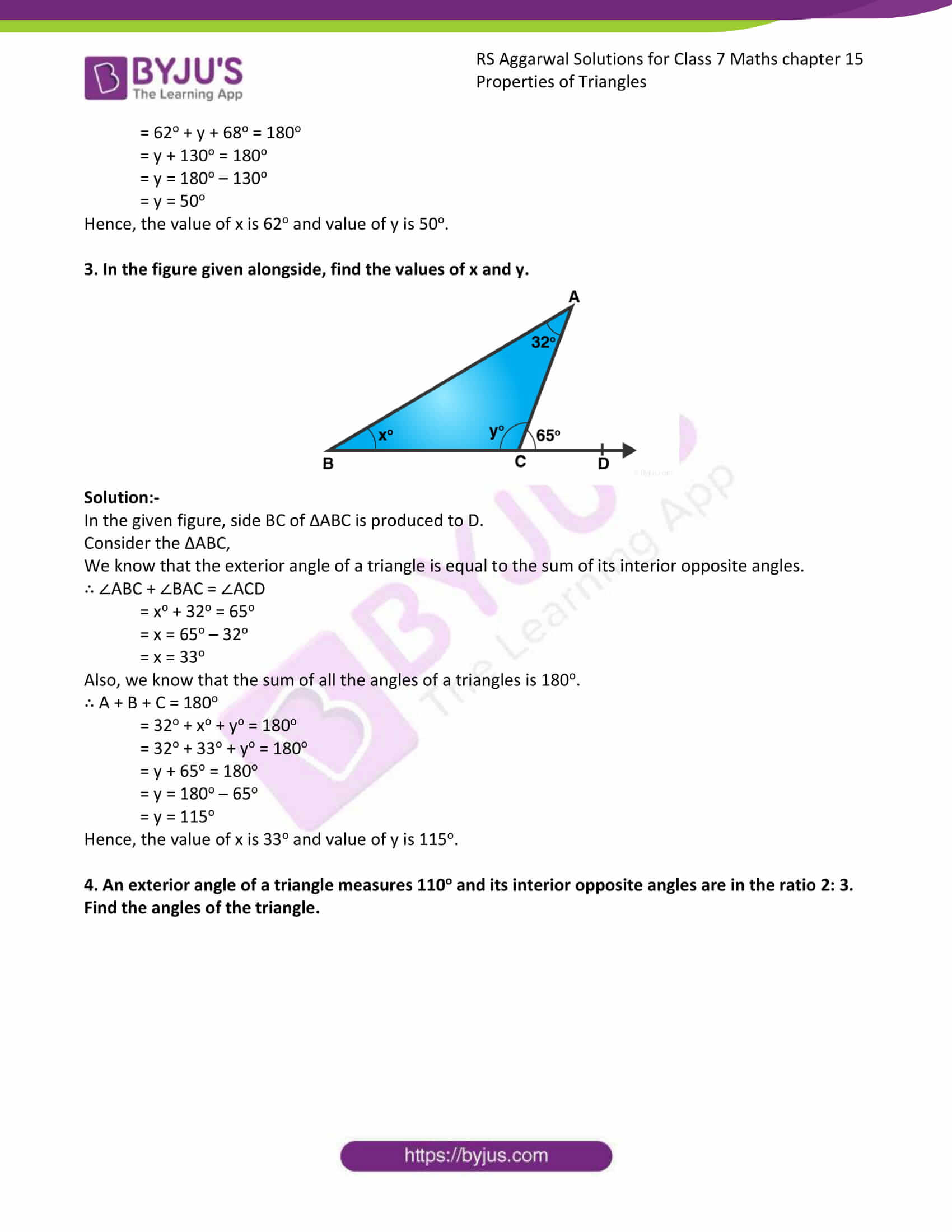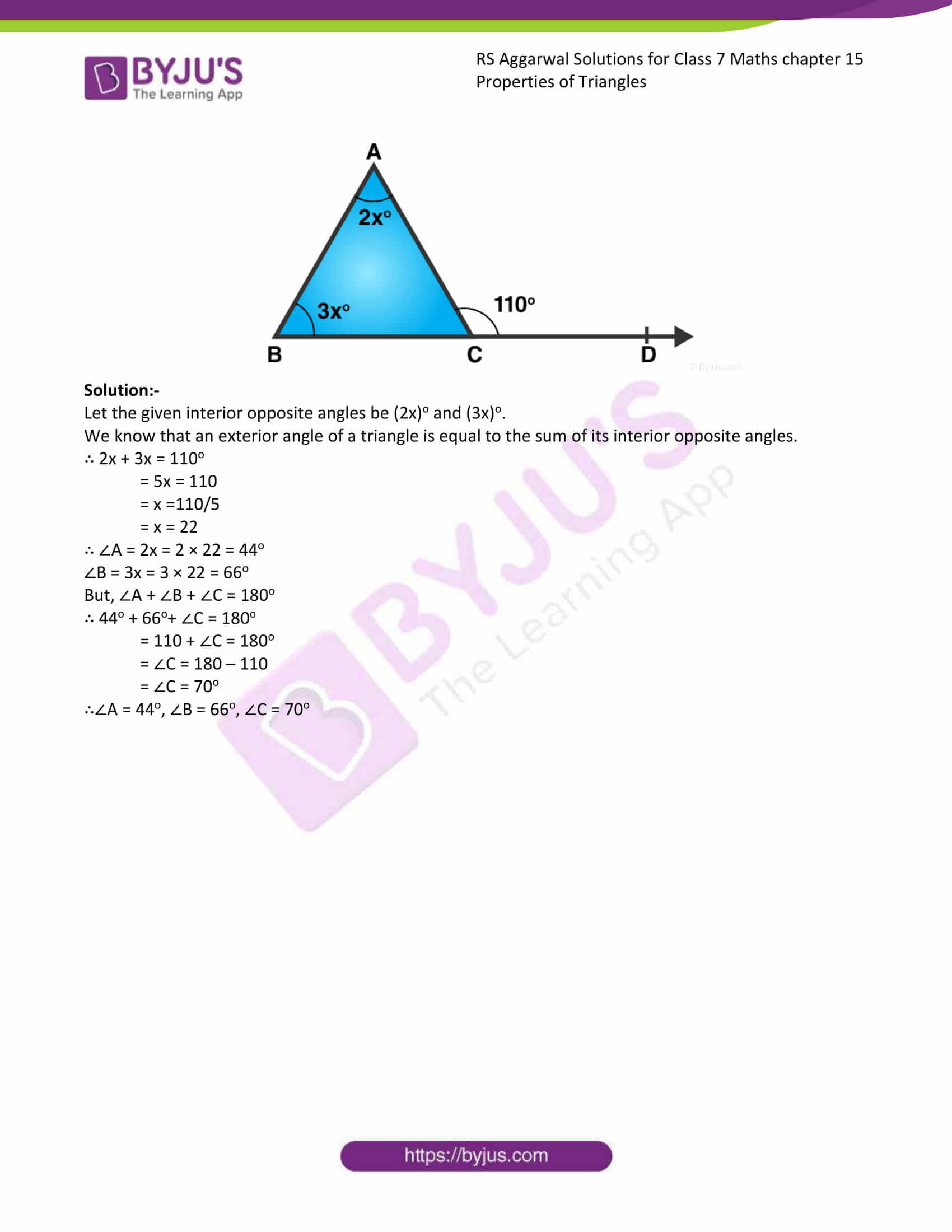### Access answers to Maths RS Aggarwal Solutions for Class 7 Chapter 15 – Properties of Triangles Exercise 15B

1. In the figure given alongside, find the measure of ∠ACD.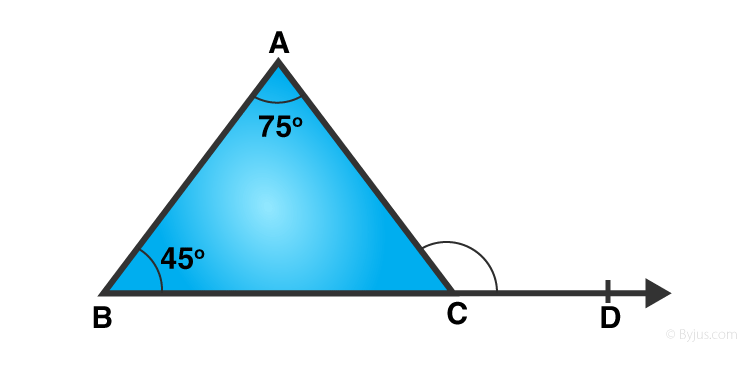Solution:-

In the given figure, side BC of ΔABC is produced to D.

Consider the ΔABC,

We know that the exterior angle of a triangle is equal to the sum of its interior opposite angles.

∴ ∠ACD = ∠ABC + ∠BAC

∠ACD = 45o + 75o

∠ACD = 120o

Hence, the measures of ∠ACD is 120o.

2. In the figure given alongside, find the values of x and y.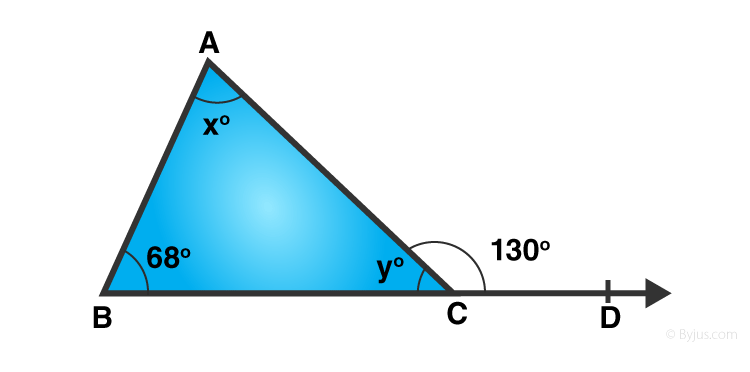Solution:-

In the given figure, side BC of ΔABC is produced to D.

Consider the ΔABC,

We know that the exterior angle of a triangle is equal to the sum of its interior opposite angles.

∴ ∠ABC + ∠BAC = ∠ACD

= 68o + x = 130o

= x = 130o – 68o

= x = 62o

Also, we know that the sum of all the angles of a triangles is 180o.

∴ x + y + 68o = 180o

= 62o + y + 68o = 180o

= y + 130o = 180o

= y = 180o – 130o

= y = 50o

Hence, the value of x is 62o and value of y is 50o.

3. In the figure given alongside, find the values of x and y.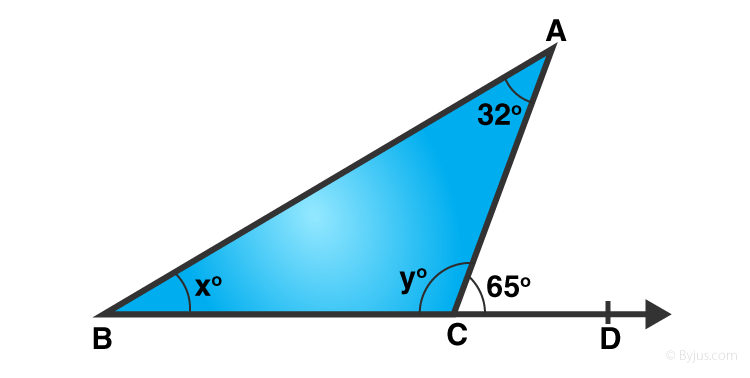Solution:-

In the given figure, side BC of ΔABC is produced to D.

Consider the ΔABC,

We know that the exterior angle of a triangle is equal to the sum of its interior opposite angles.

∴ ∠ABC + ∠BAC = ∠ACD

= xo + 32o = 65o

= x = 65o – 32o

= x = 33o

Also, we know that the sum of all the angles of a triangles is 180o.

∴ A + B + C = 180o

= 32o + xo + yo = 180o

= 32o + 33o + yo = 180o

= y + 65o = 180o

= y = 180o – 65o

= y = 115o

Hence, the value of x is 33o and value of y is 115o.

4. An exterior angle of a triangle measures 110o and its interior opposite angles are in the ratio 2: 3. Find the angles of the triangle.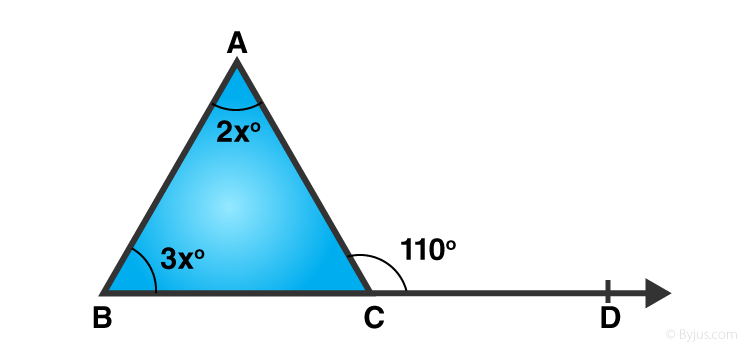Solution:-

Let the given interior opposite angles be (2x)o and (3x)o.

We know that an exterior angle of a triangle is equal to the sum of its interior opposite angles.

∴ 2x + 3x = 110o

= 5x = 110

= x =110/5

= x = 22

∴ ∠A = 2x = 2 × 22 = 44o

∠B = 3x = 3 × 22 = 66o

But, ∠A + ∠B + ∠C = 180o

∴ 44o + 66o+ ∠C = 180o

= 110 + ∠C = 180o

= ∠C = 180 – 110

= ∠C = 70o

∴∠A = 44o, ∠B = 66o, ∠C = 70o

### Access other exercises of RS Aggarwal Solutions For Class 7 Chapter 15 – Properties of Triangles

Exercise 15A Solutions

Exercise 15C Solutions

Exercise 15D Solution